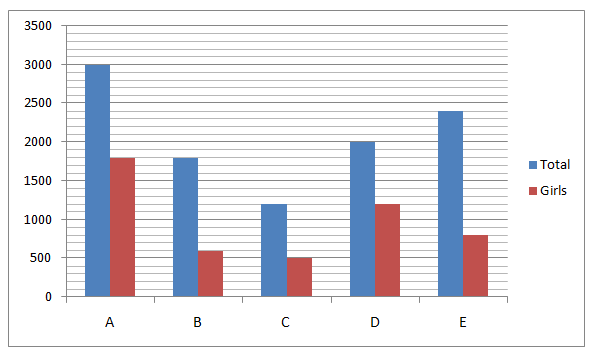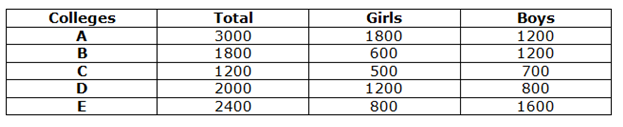# SBI PO Prelims Quantitative Aptitude Questions 2021 (Day-02)

Dear Aspirants, Our IBPS Guide team is providing new series of Quantitative Aptitude Questions for SBI PO 2021 so the aspirants can practice it on a daily basis. These questions are framed by our skilled experts after understanding your needs thoroughly. Aspirants can practice these new series questions daily to familiarize with the exact exam pattern and make your preparation effective. Added to that we have given SBI PO Prelims free practice set pdf and SBI PO Book PDF for download.

### Start Quiz

Simplification

Directions (01-05): What value should come in the place of (?) in the following questions.

1) 40 % of 300 + 15 * 24 = ? + 275

A.205

B.210

C.220

D.225

E.240

2) 19 * 37 + 56 * 18 = ?

A.1709

B.1711

C.1721

D.1701

E.1731

3) (680 ÷ √289) * 7 = ?2 + (312 ÷ 13)

A.10

B.8

C.18

D.12

E.16

4) √1444 * 22 +19 * √484 = ? * 11

A.114

B.116

C.118

D.120

E.112

5) 25 % of 560 + 65% of 180 = ?2 + 1

A.12

B.20

C.17

D.18

E.16

Bar graph

Directions (06-10): Study the following information carefully and answer the questions given below.

The given bar graph shows the total number of students and the number of girls studying in various colleges in 2018.6) The number of boys in B is what percent of the total number of students in E?

A.40%

B.50%

C.60%

D.30%

E.None of these

7) What is the average number of boys in A and C?

A.800

B.900

C.850

D.950

E.780

8) In how many colleges, the number of boys is more than that of girls?

A.1

B.3

C.2

D.4

E.None of these

9) What is the average number of boys in all the colleges together?

A.1100

B.1000

C.1200

D.1150

E.None of these

10) What is the ratio of the average number of girls in A, B and D and the average number of students in C and E?

A.2:1

B.3:4

C.4:5

D.2:3

E.None of these

### Try SBI PO Online Practice Mock Test

40 % of 300 + 15 * 24 = ? + 275

120 + 360 = ? + 275

? = 205

19 * 37 + 56 * 18 = ?

703 + 1008 = ?

? = 1711

(680 ÷ √289) * 7 = ?2 + (312 ÷ 13)

280 = ?2 + 24

16 = ?

√1444 * 22 +19 * √484 = ? * 11

38 * 22 + 22 * 19 = ? * 11

22(38 + 19) = ? * 11

? = 114

25 % of 560 + 65% of 180 = ?2 + 1

140 + 117 = ?2 + 1

? = 16

Directions (6-10) :Required percentage = 1200/2400 * 100 = 50%

Required average = (1200 + 700)/2 = 950

Required average = (1200 + 1200 + 700 + 800 + 1600)/5

= 1100

Required ratio = (1800 + 600 + 1200)/3:(1200 + 2400)/2

= 1200:1800

= 2:3

### Download SBI PO Prelims Quants Free Practice Set Day 1 PDF

#### For Day Wise SBI PO Practice Questions

Get Quantitative Aptitude Study Materials

Get All Section Study Materials